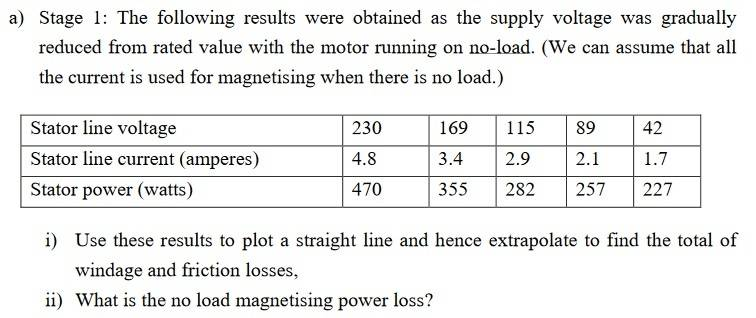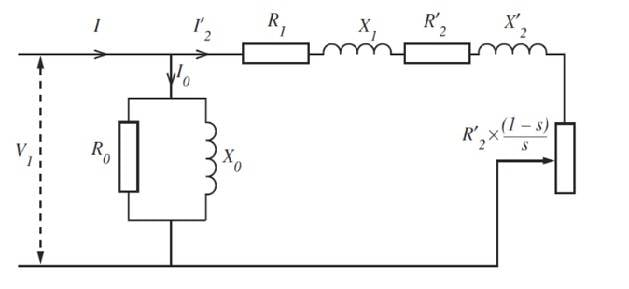# Induction motor no load magnetisation loss

• Engineering
Homework Statement:
ii) what is the no load magnetising power loss?
Relevant Equations:
R0 = V1/(Icosϕ) & X0 = V1/(Isinϕ)The study material says under 'Estimation of magnetising losses' :

On no-load, the rotor current is very small and the slip almost zero. Therefore, R′2s is very high and virtually no current flows through the branch of the circuit containing R′2s. Consequently, the no-load current is virtually equal to the current through the resistor R0 and the reactance X0, and the power taken from the supply on no-load is virtually equal to the core losses in the motor.

Therefore , R0=V1/Icosϕ and X0=V1/Isinϕ

In answering ii) using the notes:

V1 = 230
I = 4.8
ϕ = P.F (power Factor)
p = 470

P.F = P/(1.732 x V x I)

P.F = 470/(.732 x 230 x 4.8)

P.F = 0.246

using formulae from notes:

R0=V1/Icosϕ and X0=V1/Isinϕ

R0=230/(4.8cos0.246) and X0=230/(4.8sin0.246)

R0=47.92w and X0= 11160.29w

The magnetisation losses seem too large since the actual stator power is only 470w? i think i must be missing something to be getting such a large magnetisation power loss.

Henryk
Gold Member
I was told that the power factor is given as ##PF = \frac P {V*I}##. I don't understand where did you get the coefficient 1.732 (or is it 0.732??). Now, the true power is given by (in case where both, current and voltage are sinusoidal) ##P = V * I * cos(\phi)##, in other words, power factor is just ##cos(\phi)## (not ##\phi##!!)

In my opinion, the idea of this exercise is to consider the stator power as magnetic losses and windage and friction-neglecting other. Since the speed is constant the windage and friction losses are constant, also.

The magnetic losses have to be proportional with voltage. Then if voltage is "zero" only windage and friction remain. [by the way if voltage is zero no torque is expected so the rotor does not rotate!]

In this case windage and friction losses are approx.219 W [from the extension of the curve P_V] then the magnetic losses at 230 V are 251 W. From here Ro=V^2/Pmag. But Xo=3.V^2/Q [Q=total reactive power input-if S it is the apparent power=3*Vline*Iline then Q=√(S^2-Ptot^2) Ptot [at 230 V =470 W]

Last edited:
I was told that the power factor is given as PF=PV∗I. I don't understand where did you get the coefficient 1.732 (or is it 0.732??). Now, the true power is given by (in case where both, current and voltage are sinusoidal) P=V∗I∗cos(ϕ), in other words, power factor is just cos(ϕ) (not ϕ!!)
1.732 is just √3 , which is in the equation as it is a three phase supply i think. If cosϕ is power factor in this scenario im confused as to what that would make sinϕ?

In my opinion, the idea of this exercise is to consider the stator power as magnetic losses and windage and friction-neglecting other. Since the speed is constant the windage and friction losses are constant, also.

The magnetic losses have to be proportional with voltage. Then if voltage is "zero" only windage and friction remain. [by the way if voltage is zero no torque is expected so the rotor does not rotate!]

This makes sense

In this case windage and friction losses are approx.219 W [from the extension of the curve P_V] then the magnetic losses at 230 V are 251 W.
This is where i have become a bit confused. I have plotted my own curve (used to answer question i.) using the data given and i am unsure how you have managed to conclude the wining and friction losses from this graph?

#### Attachments

Calculating Ro then 218.6 W for windage and friction it is the value for which the Ro is almost constant.
V=230 Ptot=470 W Pmag=(470-218.6)=251.4 Ro=3*230^2/251.4= 631.26 ohm

#### Attachments

Calculating Ro then 218.6 W for windage and friction it is the value for which the Ro is almost constant.
V=230 Ptot=470 W Pmag=(470-218.6)=251.4 Ro=3*230^2/251.4= 631.26 ohm
Thanks for the info. I have been applying the formula and have some answers, X0 using Xo=3.V^2/Q gave me 48.41W at maximum stator voltage of 230V. I thought the inductance here would be almost equivalent to the magnetic losses , i.e. the stator power. With the formula why is the voltage squared and multiplied by 3? i am trying to relate it to the R0 and X0 formula i have but can't seem to figure it out.

The expression "line voltage" it is not clear.
According to presented schematic diagram Vl is the single phase supply voltage.
If the three stator windings are connected in Y, Vline is the voltage between phase terminal and neutral.
If the three stator windings are connected in Δ, Vline is the voltage between two phase terminals.
As 230 V it is an IEC voltage [USA it is 480, 240 , 208 or 120 V 60 Hz] it is phase-to-neutral voltage [the rated voltage is 400 V 50 Hz].
Let's say 230 V is the voltage between two phases. Then, if S it is the apparent power then S^2=P^2+Q^2.In any case S>=P.
However, the last item [42 V and 1.7 A] is less than the possible [in any case has to be 2A instead of 1.7A].
But even if it will be 2A, S remains less than P if we consider 42 V as the voltage phase-to-phase.
So, my impression is "line voltage" is phase-to-neutral voltage